Courses

# Test: Flow in Open Channel - 2

## 9 Questions MCQ Test Topicwise Question Bank for Mechanical Engineering | Test: Flow in Open Channel - 2

Description
This mock test of Test: Flow in Open Channel - 2 for Mechanical Engineering helps you for every Mechanical Engineering entrance exam. This contains 9 Multiple Choice Questions for Mechanical Engineering Test: Flow in Open Channel - 2 (mcq) to study with solutions a complete question bank. The solved questions answers in this Test: Flow in Open Channel - 2 quiz give you a good mix of easy questions and tough questions. Mechanical Engineering students definitely take this Test: Flow in Open Channel - 2 exercise for a better result in the exam. You can find other Test: Flow in Open Channel - 2 extra questions, long questions & short questions for Mechanical Engineering on EduRev as well by searching above.
QUESTION: 1

Solution:
QUESTION: 2

### The energy loss in a hydraulic jump is

Solution:

Hydraulic jump is a case of rapidly varied flow. It occurs when a super-critical flow stream tries to reach its alternate depth in subcritica! mode. In the process it losses substantial energy and falls short of the alternate depth.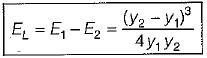QUESTION: 3

### What is the velocity of flow of water through a rectangular channel of 6 m wide and 3 m deep, When it is running full. The channel is having bed slope as 1 in 2000. [Take Chezy’s constant C = 55]

Solution:

b = 6 m, d = 3 m
A = b x d = 18 m2,
P = b + 2d - 6 + 6 = 12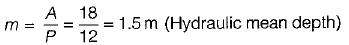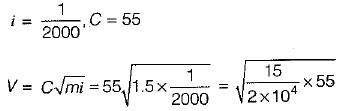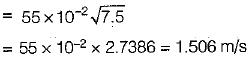[Tips: This type of question can be solved by guessing about square root i.e. 2.42 = 5.76,2.62 = 6.76, 2.82 = 7.84].

QUESTION: 4

How is the best hydraulic channel cross-section defined?

Solution:
QUESTION: 5

A rectangular channel with be most economical when the flow depth to bottom width are in the ratio

Solution: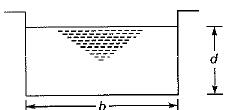For minimum wetted perimeter,
d = b/2
∴ d/b = 1.2

QUESTION: 6

In open channel flow, a triangular section will be most economical when the vertex angle at the triangle base point is

Solution:
QUESTION: 7

The discharge through a trapezoidal channel is maximum when the top width equals

Solution:
QUESTION: 8

The best slope for the most economical trapezoidal section of an open channel is ..... to the horizontal.

Solution: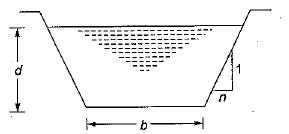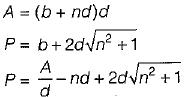for best side slope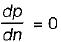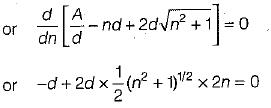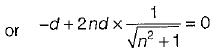or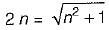or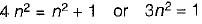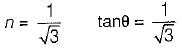or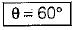QUESTION: 9

Most efficient channel section is

Solution: